# Samacheer Kalvi 6th Maths Solutions Term 1 Chapter 3 Ratio and Proportion Ex 3.2

## Tamilnadu Samacheer Kalvi 6th Maths Solutions Term 1 Chapter 3 Ratio and Proportion Ex 3.2Question 1.
Fill in the blanks of the given equivalent ratios.
(i) 3 : 5 = 9 : ___
(ii) 4 : 5 = ___ : 10
(iii) 6 : ____ = 1 : 2
Solution:
(i) 15
Hint: $$\frac{3}{5}=\frac{3 \times 3}{5 \times 3}=\frac{9}{15}$$
(ii) 8
Hint: $$\frac{4}{5}=\frac{4 \times 2}{5 \times 2}=\frac{8}{10}$$
(iii) 12
Hint: $$\frac{1}{2}=\frac{1 \times 6}{2 \times 6}=\frac{6}{12}$$

Question 2.
Complete the Table: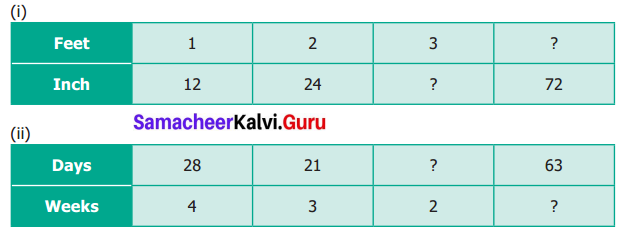Solution: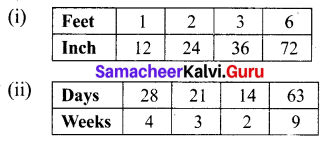Question 3.
Say True or False.
(i) 5 : 7 is equivalent to 21 : 15.
(ii) If 40 is divided in the ratio 3 : 2, then the larger part is 24.
Solution:
(i) False
(ii) True

Question 4.
Give two equivalent ratios for each of the following.
(i) 3 : 2
(ii) 1 : 6
(iii) 5 : 4
Solution: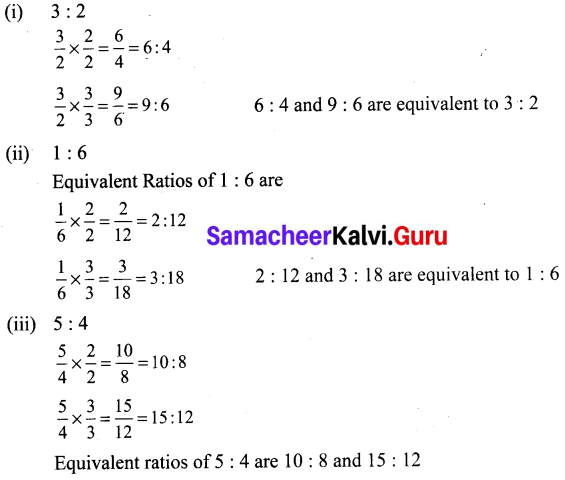Question 5.
Which of the two ratios is larger?
(i) 4 : 5 or 8 : 15
(ii) 3 : 4 or 7 : 8
(iii) 1 : 2 or 2 : 1
Solution: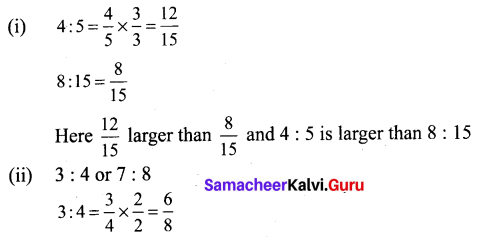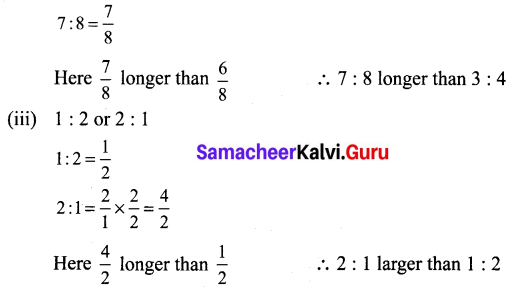Question 6.
Divide the numbers given below in the required ratio.
(i) 20 in the ratio 3 : 2
(ii) 27 in the ratio 4 : 5
(iii) 40 in the ratio 6 : 14
Solution:
(i) Ratio = 3 : 2
Sum of the ratio = 3 + 2 = 5
5 parts = 20
1 part = $$\frac{20}{5}$$
= 4
3 parts = 3 × 4 = 12
2 parts = 2 × 4 = 8
20 can be divided in the form as 12, 8.

(ii) Ratio = 4 : 5
Sum of the ratio = 4 + 5 = 9
9 parts = 27
1 part = $$\frac{27}{9}$$ = 3
4 parts = 4 × 3 = 12
5 parts = 5 × 3 =15
27 can be divided in the form as 12, 15.

(iii) 40 in the ratio 6 : 14
Ratio = 6 : 14
Sum of the ratio = 6 + 14 = 20
20 parts = 40
1 part = $$\frac{40}{20}$$ = 2
6 parts = 2 × 6 = 12
14 parts = 2 × 14 = 28
40 can be divided in the form as 12, 28.

Question 7.
In a family, the amount spent in a month for buying Provisions and Vegetables are in the ratio 3 : 2. If the allotted amount is ₹ 4000, then what will be the amount spent for
(i) Provisions and
(ii) Vegetables?
Solution:
Dividing the total amount ₹ 4000 into 3 + 2 = 5 equal parts then
(i) For Provisions:
3 out of 5 parts are spent for provisions and 2 out of 5 parts for vegetables.
$$4000 \times \frac{3}{5}=2400$$ for provisions
(ii) For vegetables:
$$4000 \times \frac{2}{5}=1600$$ for Vegetables.
₹ 2400 spend on provisions and ₹ 1600 spend on Vegetables.Question 8.
A line segment 63 cm long is to be divided into two parts in the ratio 3 : 4. Find the length of each part.
Solution:
Total length = 63 cm Ratio = 3 : 4
Sum of the ratio = 3 + 4 = 7
7 parts = 63 cm
1 part = $$\frac{63}{7}$$ = 9 cm
3 parts = 3 × 9 cm = 27 cm
4 parts = 4 × 9 cm = 36 cm
∴ 63 cm can be divided into the parts as 27 cm and 36 cm.

Objective Type Questions

Question 9.
If 2 : 3 and 4 : ___ or equivalent ratios, then the missing term is ____
(a) 6
(b) 2
(c) 4
(d) 3
Solution:
(a) 6
Hint: $$\frac{2}{3}=\frac{2 \times 2}{3 \times 2}=\frac{4}{6}$$

Question 10.
An equivalent ratio of 4 : 7 is
(a) 1 : 3
(b) 8 : 15
(c) 14 : 8
(d) 12 : 21
Solution:
(d) 12 : 21

Question 11.
Which is not an equivalent ratio of $$\frac{16}{24}$$ ?
(a) $$\frac{6}{9}$$
(b) $$\frac{12}{18}$$
(c) $$\frac{10}{15}$$
(d) $$\frac{20}{28}$$
Solution:
(d) $$\frac{20}{28}$$
Hint: $$\frac{16}{24}=\frac{8 \times 2}{8 \times 3}=\frac{2}{3}$$

Question 12.
If Rs 1600 is divided
(a) Rs 480
(b) Rs 800
(c) Rs 1000
(d) Rs 200
Solution:
(c) Rs 1000